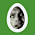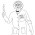## Thursday, March 17, 2016

### Putting that Math degree to work. From Nick.

Otherwise known as "Fermat's Last Theorem," this equation was first posed by French mathematician Pierre de Fermat in 1637, and had stumped the world's brightest minds for over 300 years.

In the 1990s, Oxford professor Andrew Wiles finally solved the problem, and this week was awarded the hugely prestigious 2016 Abel Prize -- including a \$700,000 windfall.

http://www.cnn.com/2016/03/16/europe/fermats-last-theorem-solved-math-abel-prize/?iid=ob_homepage_NewsAndBuzz_pool&iref=obinsite

1.I think it's x^n + y^n = z^n. It had me scratching my head what all the fuss was about until I clicked the link.

1.The theorem states that x^n + y^n = z^n has no solutions for integers x,y and z (apart from trivial cases, like x=0 and y=z) for any integer n>2. So the summary is reasonable: x^n + y^n =/= z^n for any n>2. When n=2 there are many infinitely many solutions, known as pythagorean triples (for example, 3^2+4^2=5^2).

2.Why did it take 20 years to get his money together?

1.Maybe it came from an anonymous account.

2.If scientists were paid as well as professional athletes, there would be no disease.